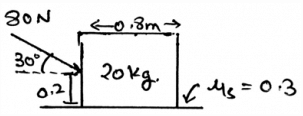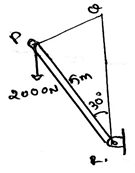Test: Rolling Resistance

# Test: Rolling Resistance

Test Description

## 15 Questions MCQ Test Engineering Mechanics | Test: Rolling Resistance

Test: Rolling Resistance for Mechanical Engineering 2022 is part of Engineering Mechanics preparation. The Test: Rolling Resistance questions and answers have been prepared according to the Mechanical Engineering exam syllabus.The Test: Rolling Resistance MCQs are made for Mechanical Engineering 2022 Exam. Find important definitions, questions, notes, meanings, examples, exercises, MCQs and online tests for Test: Rolling Resistance below.
Solutions of Test: Rolling Resistance questions in English are available as part of our Engineering Mechanics for Mechanical Engineering & Test: Rolling Resistance solutions in Hindi for Engineering Mechanics course. Download more important topics, notes, lectures and mock test series for Mechanical Engineering Exam by signing up for free. Attempt Test: Rolling Resistance | 15 questions in 30 minutes | Mock test for Mechanical Engineering preparation | Free important questions MCQ to study Engineering Mechanics for Mechanical Engineering Exam | Download free PDF with solutions
 1 Crore+ students have signed up on EduRev. Have you?
Test: Rolling Resistance - Question 1

### What does the moment of the force measure in the rolling of the body?

Detailed Solution for Test: Rolling Resistance - Question 1

The moment of the force measures the tendency of the rotation of the body along any axis, whether it be the centroid axis of the body, or any of the outside axis. The couple moment is produced by two forces, not by a single force. The total work done is the dot product of force and distance not the cross.

Test: Rolling Resistance - Question 2

### Determine the vertical force acting in the given figure.Detailed Solution for Test: Rolling Resistance - Question 2

The net forces acting on the body is shown by the help of the resultant forces. There are two types, first the frictional and the second is the normal. This is because the resultant forces have the sum of all the forces which are acting on the direction which is same.

Test: Rolling Resistance - Question 3

### If a car is moving forward, what is the direction of the moment of the moment caused by the rolling of the tires, assume non slippery surface?

Detailed Solution for Test: Rolling Resistance - Question 3

When you curl your wrist in the direction in which the tires are moving then you will find that the thumb is pointing outwards. That is outwards the body of the car. This phenomena is also observed in rainy seasons. When cars travel on the roads, the water is thrown outside from the tires, due to moment.

Test: Rolling Resistance - Question 4

The moment of the force is the product of the force and the perpendicular distance of the axis and the point of action of the force. Is this also true for rolling?

Detailed Solution for Test: Rolling Resistance - Question 4

The moment is the product of the force applied to the body and the perpendicular distance of the point of action of the force to the axis about which the body is being rotated. That is the moment is the cross product of the force and the distance between the axis and the point of action. Yes this is also true for rolling.

Test: Rolling Resistance - Question 5

For the rolling of the body the calculation of the moment of the force about the axis of rolling, the cross product table, i.e. the 3X3 matrix which is made for doing the cross product having 3 rows, contains three elements. Which are they from top to bottom?

Detailed Solution for Test: Rolling Resistance - Question 5

The 3X3 matrix which is being made is having axis coordinates, point coordinates and the force coordinates. They are from top to bottom placed. The order cannot be changed. Or if changed then one needs to apply the negative sign appropriately. Negative because the directions gets reversed.

Test: Rolling Resistance - Question 6

Find the moment of the force about the point R.Detailed Solution for Test: Rolling Resistance - Question 6

As we know that the moment is the cross product of the distance and the force we will try to apply the same here. We see that the perpendicular distance is 5m. Thus we get the distance. And hence multiply it with the force, 5x2000xsin30. Because the force component perpendicular to the distance need to be taken.

Test: Rolling Resistance - Question 7

In rolling there is the involvement of the vector math. So for rolling which of the following is correct? (For A representing the vector representation of the axis of rotation, r the radius vector and F the force vector)

Detailed Solution for Test: Rolling Resistance - Question 7

The correct form of the equation is given by A.(rxF). Where A represents the vector representation of the axis of rotation, r the radius vector and F the force vector. This is usually done for determining the moment of the force about the axis. That is if body is being rotated by the force about an axis.

Test: Rolling Resistance - Question 8

In the equation A.(rxF), the r vector is what?

Detailed Solution for Test: Rolling Resistance - Question 8

The r in the equation A.(rxF) is the radius vector of the rolling body. That is it is the vector which is having the start point at the axis of rolling and the end point at the point of action of the force on the rolling body. This vector is being crossed by the force vector, which is then followed by the dot product with the axis vector.

Test: Rolling Resistance - Question 9

The ___________ forces do not cause the rotation/rolling of the body if the rotation is considered in about the axis of the body or the centroid axis of the body.

Detailed Solution for Test: Rolling Resistance - Question 9

The concurrent forces are the which are somewhere touching the axis of rotation. If any of the force is touching that axis, that force is not considered, or is insufficient to cause a rotation. If a force is concurrent then the perpendicular distance of the force from the line of axis is zero, thus no rotation. As we know rotation is caused by moment.

Test: Rolling Resistance - Question 10

For the rolling of the body right handed coordinate system means (consider the mentioned axis to be positive)?

Detailed Solution for Test: Rolling Resistance - Question 10

As right handed coordinate system means that you are curling your fingers from positive x-axis towards y-axis and the thumb which is projected is pointed to the positive z-axis. Thus visualizing the same and knowing the basic members of axis will not create much problem. The right handed coordinate system is universal throughout.

Test: Rolling Resistance - Question 11

In the equation Wa/r generally used in the rolling frictional calculations, what does each stands for?

Detailed Solution for Test: Rolling Resistance - Question 11

The rolling of the cylinder is termed as perfect rolling only when the cylinder is rolling at a constant velocity. Also the rolling should be along the rigid surface. The normal forces are acting perpendicular to the surface of the rolling. And for rolling equation Wa/r is generally used.

Test: Rolling Resistance - Question 12

Which statement is correct about the vector F acting parallel to the direction of the motion of the rolling body?

Detailed Solution for Test: Rolling Resistance - Question 12

As we know the α, β and γ are the angles made by the x, y and z-axis respectively. Thus, is the magnitude of the vector is F, the F= Fcos β + Fcos α + Fcosγ. Which means the force is the resultant of all its axis’ components. The force driving the rolling motion of the body can be divided like this.

Test: Rolling Resistance - Question 13

What if the moment of the force calculated about the axis of rolling is negative?

Detailed Solution for Test: Rolling Resistance - Question 13

It means that the direction of the rolling is in the opposite sense as imagined. We can’t say about the direction of the force or the direction of the radius vector. But yes we can say about the direction of the rolling as it is the thing which is going to be calculated. Rest all the parts are fixed. They can’t be altered.

Test: Rolling Resistance - Question 14

In the equation A.(rxF) the r is heading from ______________ and ending at _____________

Detailed Solution for Test: Rolling Resistance - Question 14

It is the radius vector. The radius vector is always from the axis of rolling to the point of action of the force on the body. Which means that the radius vector is not on any point on the force vector. Rather it ending at the point on the force vector, where it is being in contact of the body.

Test: Rolling Resistance - Question 15

Rotation is termed as rolling when _______

Detailed Solution for Test: Rolling Resistance - Question 15

The rolling of the cylinder is termed as perfect rolling only when the cylinder is rolling at a constant velocity. Also the rolling should be along the rigid surface. The normal forces are acting perpendicular to the surface of the rolling.

## Engineering Mechanics

24 videos|71 docs|39 tests
 Use Code STAYHOME200 and get INR 200 additional OFF Use Coupon Code
Information about Test: Rolling Resistance Page
In this test you can find the Exam questions for Test: Rolling Resistance solved & explained in the simplest way possible. Besides giving Questions and answers for Test: Rolling Resistance, EduRev gives you an ample number of Online tests for practice

## Engineering Mechanics

24 videos|71 docs|39 tests